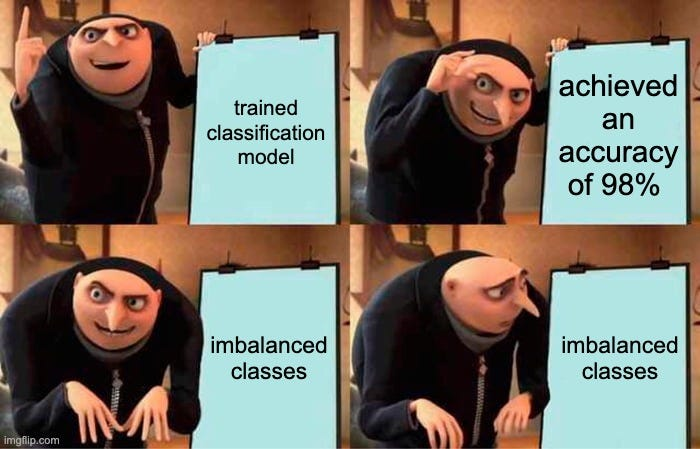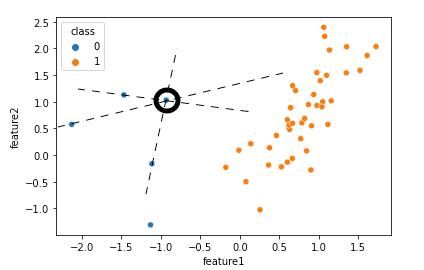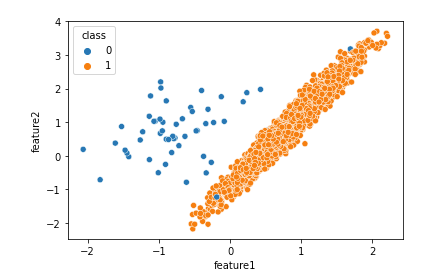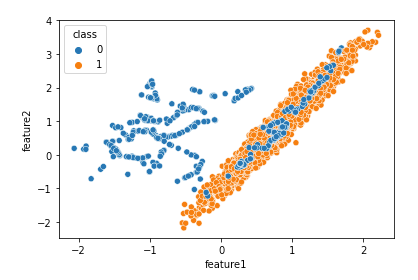# Quick Guide to Deal with Imbalanced DataMeme by author | Generated through https://imgflip.com/memegenerator | Photo source belongs to Universal Pictures — Despicable Me movies

We are living in interesting times as data scientists. We have seen the exponential growth of cloud technologies and AutoML. All of a sudden, everyone can be a data scientist — it is no longer necessary for someone to have an MSc or Ph.D. degree unless the role involves specific novel problems. While there is a lower barrier of entry now to the field, I strongly believe as data scientists, we must master the nitty-gritty of at the very least, classical machine learning algorithms as well as a few technical concepts.

From my experience as a data scientist, I find imbalanced classes to be one of the most underrated technical concepts, especially for new data scientists. In this article, we will address why imbalanced classes are a problem and also a few solutions that I find helpful.

## The Crux of Problem

To understand why imbalanced classes are a problem, we must go back to the fundamentals. How does a machine learning algorithm work? All machine learning algorithms, regardless of how fancy they are, aim to minimise cost functions. Since algorithms are well just algorithms, they have no notion of right and wrong unlike us. As such we use the cost functions during the training process to allow the algorithms to learn about the concept. Different algorithms will then have their own ways to minimise the functions such as for example gradient descent for linear models and backpropagation for neural networks.

In simple terms, it means all ML algorithms seek to minimise the error in their predictions. While this is a no-brainer fact, it becomes a problem when there are imbalanced classes in a dataset. Consider a scenario where we want to create a model that predicts fraudulent activities which happen sparingly (I know a very cliche example). Let's say in a dataset of 1000 observations, there are only 20 positive values (fraudulent) and 980 negative values (non-fraudulent). To yield good performance, the ML algorithms can just classify all observations as non-fraudulent (even ML algorithms hate to be wrong!)

By using Accuracy as a measure of performance, we will get:

(0 + 980) / 1000 = 0.98 accuracy!

This looks impressive but very misleading. If the objective of the model is to predict fraudulent activities, then this particular model is absolute garbage because it does not classify fraudulent activities correctly at all. The misunderstanding will get worse the more imbalanced the classes are. The more experience data scientists among you will realise that measuring performance of ML models in the presence of imbalanced classes through Accuracy is a poor choice in the first place. And you are right!

Oftentimes, the “hotfix” will be to choose more appropriate performance metrics such as recall that minimises false negatives to name a few. The hotfix however will only help us to get a better view of the effects that imbalanced classes have on the models and is only the first step. In our example above, the model will get a recall score of:

0 / (0 + 20) = 0

because it classifies none of the fraudulent activities correctly. We can also use stratified sampling before training the ML algorithms to get a more accurate view of the performance in presence of imbalanced data. The ideal solution to the imbalance problem, however, will be to collect more data such that the minority classes are more or less equal with the majority. But, in real projects, oftentimes this is not viable because of either time restrictions or the data collection process is simply too costly. So what can we do?

## Solution 1 — Threshold Classification

One thing that we can do is define custom probability thresholds for the trained models. This is one of the simplest yet overlooked methods. The output from ML models is either probabilities or some scores that indicate class membership. The bottom line is, the output will always be continuous values even in the case of classification. How then do our models output labels? or classes? The answer is the decision threshold.

Again consider our fraudulent activities example where there are two classes. One natural decision threshold will be to put it at 0.5. For e.g., if the probability of an observation is less than 0.5, we will classify it as fraudulent activities and if not, it will be classified as non-fraudulent activities. The .predict() method from scikit-learn package, for example, uses this default value for binary classification. This is again sub-optimal or downright wrong when dealing with an imbalance dataset. One thing that we can do is to “move” the decision threshold from 0.5 to some other values. To find the right decision threshold, we can either:

• consult with a subject matter expert or
• use grid search over the probability range (between 0 and 1)

To implement this using scikit-learn, use the .predict_proba() method to output probabilities instead of labels.

## Solution 2 — Sampling algorithms

The second solution is to create a more balanced dataset. While the data collection process is expensive, we can use sampling algorithms instead. For example, we can use bootstrapping and its variation to produce more of the minority classes. However, one weakness of bootstrapping is that it reduces the variation of the data which can potentially cause the models not to generalise well. My personal favourite is to use a combination of SMOTE (over-sampling algorithm)¹ and Tomek’s Links² (under-sampling algorithm). These two methods must be used together to produce the best result. To understand why we will explore both algorithms on a high level.

SMOTE

Synthetic Minority Over-sampling Technique (SMOTE) is a technique to generate synthetic data. This particular algorithm works on the “feature” space instead of the “data” space. It means SMOTE is used on data that has been preprocessed and is ready to be fed into the ML algorithms. SMOTE uses the concept of k-nearest neighbours and hence, we must determine the number of neighbours beforehand. The default value of the number of neighbours is 5. To generate the synthetic data, the following equation where x is a data point under investigation and n is its neighbour:

On a high level, the equation means we are just adding noises to the original data points. There is a small caveat though. By using the formula above, the synthetic data points will only be generated along straight lines between the data point under investigation and its neighbours. The following plot can be used for illustration:Image by Author | generated using scikit-learn python library

Suppose we use SMOTE algorithm with 3 nearest neighbours. For a particular data point, we will then identify the 3 nearest neighbours for the minority class using a distance metric. Depending on the rate of the oversampling, we will select random neighbours out of the 3 nearest neighbours that we have identified and create synthetic data (one data point for each line) along the lines. The rate of the oversampling is dependent on the number of k nearest neighbours chosen. For example, if we use the default value of 5 nearest neigbours, we can increase the data points of the minority class as many as 500% of their initial number.

This technique is better than normal bootstrapping because it adds variation to the synthetic data. At the same time, it must be used together with an undersampling algorithm, particularly Tomek’s Links because otherwise, it will “blur” and shift the decision boundary.Before SMOTE | Image by Author | Generated using scikit-learn packageAfter SMOTE | Image by Author | Generated using imbalanced-learn package

As we can see, after SMOTE algorithm is used, the decision boundary that separates the two classes is less clear because more blue points are mixed with the orange points. This may yield sub-optimal ML models. To mitigate the problem, Tomek’s Links can be utilised.

A Tomek’s link between two data points of different classes is defined such that for any sample z,

In layman’s terms, two data points have a tomek’s link, if they belong to different classes and are nearest neighbours of each other. The aim is to find points along the decision boundary. The following plot can be used as illustration: